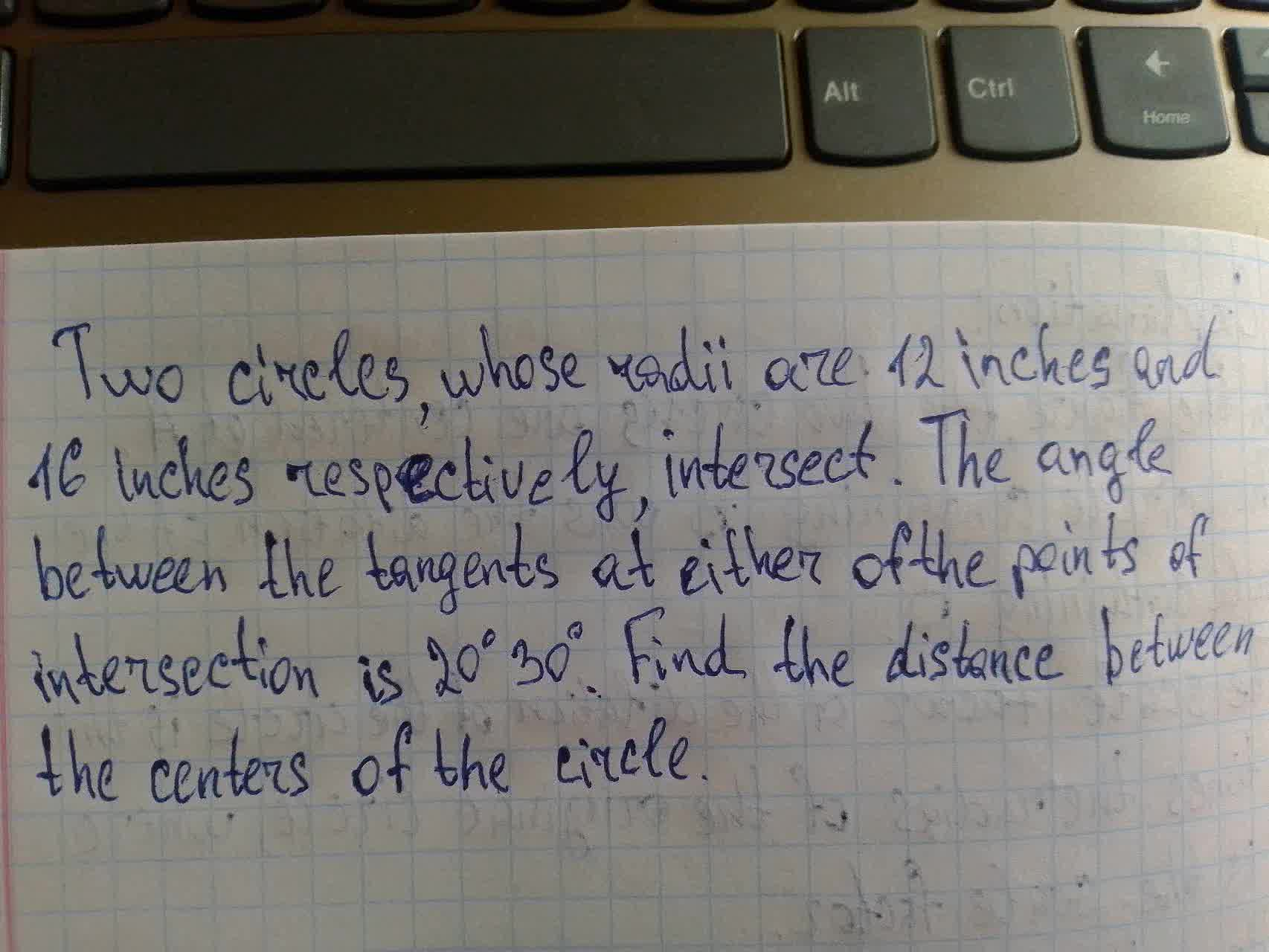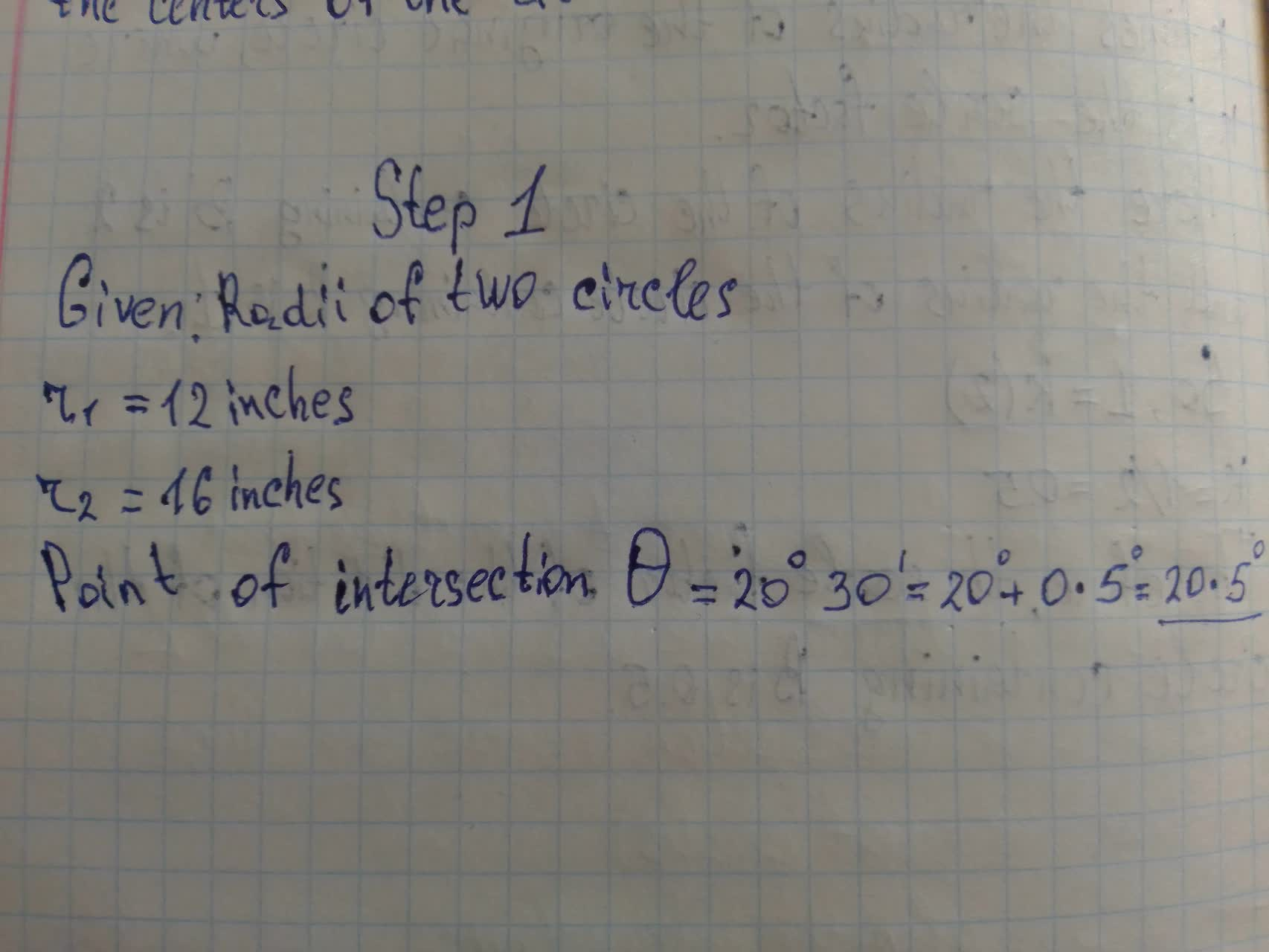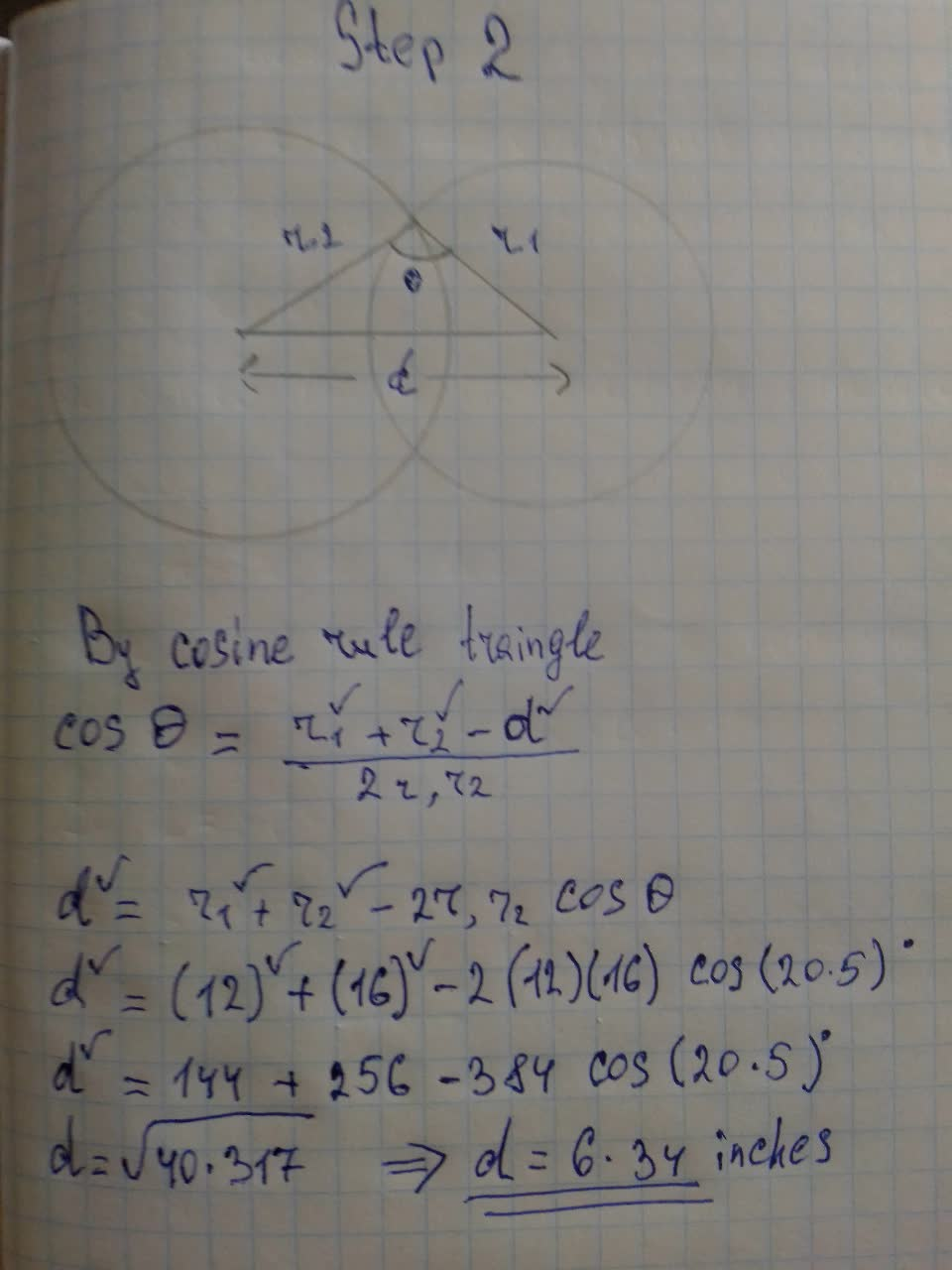Question# Two circles, whose radi are 12 inches and 16 inches respectively. intersect. The angle between the tangents at either of the points of intersection is

Circles
ANSWEREDTwo circles, whose radi are 12 inches and 16 inches respectively. intersect. The angle between the tangents at either of the points of intersection is